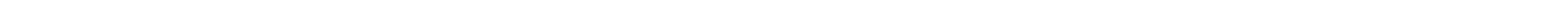Algorithms and Complexity Group

We are a group of theoretical computer scientists who are interested in a number of research areas. These areas mainly include:

Algorithmic Graph Theory
Complexity of graph algorithms, approximation and randomized algorithms in graphs.

Complexity and Constraint Satisfaction Problems
Complexity theory such as circuit complexity, pseudo-randomness, cryptography, and complexity of constraint problems.

Combinatorial Optimization
Approximation algorithms, large scale combinatorial optimization problems (in transportation, logistics and scheduling), and heuristics approaches for solving NP-hard problems.